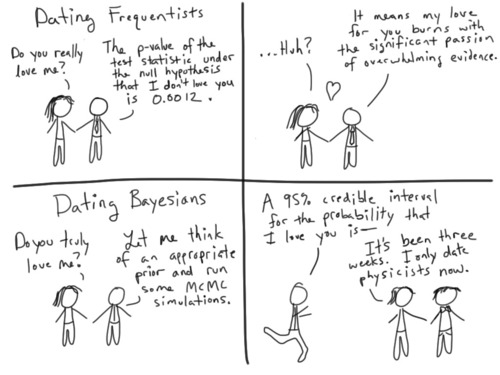Intro Research Teaching Extra

## NMST434 Modern statistical methods (official information)

Exercise class: Tuesday 17:20 - 18:50 K3 (S. Nagy)

Assumed knowledge:

The knowledge of the statistics and probability theory at the level of courses Mathematical Statistics 1 and 2 (NMSA331 and NMSA332), Probability Theory 1 (NMSA333) and Linear regression (NMSA407).

Among others we will use the following concepts: almost sure convergence, convergence in probability, convergence in distribution, law of large numbers and central limit theorem for independent and identically distributed random vectors. A nice overview (in Czech language) of most of the results that are used in the course is available here.

(Assumed) content of the course:
• Asymptotic methods - Delta Theorem, Moment estimators
• Theory of maximum likelihood
• Profile, conditional and marginal likelihood
• M-estimators and Z-estimators, Robust estimation
• Bootstrap
• Quantile regression
• EM-algorithm
• Methods for missing data
• Kernel density estimation
• Kernel nonparametric regression

Lecture material and actual information